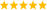# LabVIEW

cancel
Showing results for
Did you mean:

Solved!
Go to solution

## Subtract the mean of a matrix from each element

Hi,

I am doing principle component analysis I have an original real matrix feeding in to a the covarience matrix which gives the mean out at one of the nodes and want to subtract that from each element of the original matrix to form a new matrix. If this is to hard to connect the mean out of the covariance matrix is there a way of just finding the mean of the original matrix and subtracting this from each element in the matrix to form a new matrix.

Regards

Canalian

Message 1 of 5
(1,425 Views)

## Re: Subtract the mean of a matrix from each element

The Subtract primitive on the Numeric palette is polymorphic.  You can subtract a scalar from a matrix or an array.  The result is also a matrix or an array with the scalar value subtracted from each element.

Lynn

Message 2 of 5
(1,413 Views)

## Re: Subtract the mean of a matrix from each element

Hi Lynn,

Thanks for your quick reply. I must have been tired last night I realised that the problem is that I have a 1d matrix comming out of the covariance matrix and I am trying to subtract that from a each element of a 2d matrix, but I don know how to do this. I have attached the code of what I am trying showin the broken wire.

Regards

Nick

Message 3 of 5
(1,401 Views)
Solution
Accepted by topic author Canalian

## Re: Subtract the mean of a matrix from each element

The mean of a 2D array is simply the sum of all elements divided by the number of elements (=the product of the dimensions).

All you need is the following (works equally well for arrays with more than 2 dimensions):LabVIEW Champion. It all comes together inGCentralMessage 4 of 5
(1,396 Views)

## Re: Subtract the mean of a matrix from each element

Thanks that is really appreciated

Regards

Nick

Message 5 of 5
(1,389 Views)